# 27 Results

View
Selected filters:
• CCSS.Math.Content.HSA-CED.A.2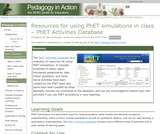Conditional Remix & Share Permitted
CC BY-NC-SA
Rating

The PhET Activities Database is a collection of resources for using PhET sims. It includes hundreds of lesson plans, homework assignments, labs, clicker questions, and more. Some activities have been created by the PhET team and some have been created by teachers.

Subject:
Life Science
Physical Science
Material Type:
Activity/Lab
Simulation
Provider:
Science Education Resource Center (SERC) at Carleton College
Provider Set:
Pedagogy in Action
Starting Point (SERC)
Author:
Sam McKagan
08/28/2012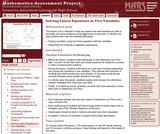Only Sharing Permitted
CC BY-NC-ND
Rating

This lesson unit is intended to help teachers assess how well students are able to formulate and solve problems using algebra and, in particular, to identify and help students who have the following difficulties: solving a problem using two linear equations with two variables; and interpreting the meaning of algebraic expressions.

Subject:
Algebra
Material Type:
Assessment
Lesson Plan
Provider:
Shell Center for Mathematical Education
U.C. Berkeley
Provider Set:
Mathematics Assessment Project (MAP)
04/26/2013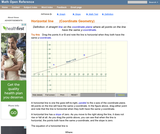Rating

An interactive applet and associated web page that show the definition of a horizontal line in coordinate geometry. The applet has two points that the user can drag which define a line. The line flagged when it is horizontal (slope=0) and the equation of the line is shown. The grid, details and coordinates can be turned on and off. The applet can be printed exactly as it appears on the screen to make handouts. The web page has a discussion on how to test for horizontal, the line equation and has links to other pages relating to coordinate geometry. Applet can be enlarged to full screen size for use with a classroom projector. This resource is a component of the Math Open Reference Interactive Geometry textbook project at http://www.mathopenref.com.

Subject:
Geometry
Material Type:
Simulation
Provider:
Math Open Reference
Author:
John Page
02/16/2011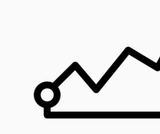Conditional Remix & Share Permitted
CC BY-NC-SA
Rating

This resource was created by the Washington Office of Superintendent of Public Instruction.

Subject:
Mathematics
Material Type:
Assessment
Homework/Assignment
Lesson
Author:
Hannah Hynes-Petty
03/31/2020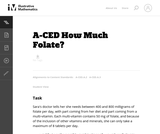Unrestricted Use
CC BY
Rating

This is a task from the Illustrative Mathematics website that is one part of a complete illustration of the standard to which it is aligned. Each task has at least one solution and some commentary that addresses important asects of the task and its potential use. Here are the first few lines of the commentary for this task: Sara's doctor tells her she needs between 400 and 800 milligrams of folate per day, with part coming from her diet and part coming from a multi-vitamin...

Subject:
Mathematics
Material Type:
Activity/Lab
Provider:
Illustrative Mathematics
Provider Set:
Illustrative Mathematics
Author:
Illustrative Mathematics
05/27/2013Unrestricted Use
CC BY
Rating

This is a task from the Illustrative Mathematics website that is one part of a complete illustration of the standard to which it is aligned. Each task has at least one solution and some commentary that addresses important aspects of the task and its potential use.

Subject:
Algebra
Material Type:
Activity/Lab
Provider:
Illustrative Mathematics
Provider Set:
Illustrative Mathematics
Author:
Illustrative Mathematics
08/06/2015Unrestricted Use
CC BY
Rating

This question examines the algebraic equations for three different spheres. The intersections of each pair of spheres are then studied, both using the equations and thinking about the geometry of the spheres. For two spheres where one is not contained inside of the other there are three possibilities for how they intersect.

Subject:
Mathematics
Algebra
Geometry
Material Type:
Activity/Lab
Provider:
Illustrative Mathematics
Provider Set:
Illustrative Mathematics
Author:
Illustrative Mathematics
01/21/2013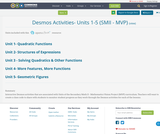Conditional Remix & Share Permitted
CC BY-SA
Rating

Interactive Desmos activities that are associated with Units of the Secondary Math II - Mathematics Vision Project (MVP) curriculum.
Teachers will want to create a class code to share with students to monitor student progress as they work through the Desmos activities for each of the lessons.

Subject:
Mathematics
Material Type:
Interactive
Author:
Mindy Branson
10/24/2019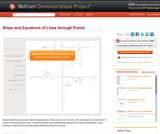Conditional Remix & Share Permitted
CC BY-NC-SA
Rating

Algebra students need practice determining equations of lines given a pair of points, or the line parallel or perpendicular to a given line through a given point. This Demonstration, along with guiding worksheets or a teacher presentation, gives students a chance to see the relationships between these lines and points.

Subject:
Algebra
Material Type:
Activity/Lab
Diagram/Illustration
Homework/Assignment
Interactive
Simulation
Teaching/Learning Strategy
Provider:
Wolfram Research
Provider Set:
Wolfram Demonstrations Project
Author:
Tom Falcone
09/04/2013Conditional Remix & Share Permitted
CC BY-NC-SA
Rating

This resource was created by the Washington Office of Superintendent of Public Instruction.

Subject:
Mathematics
Material Type:
Assessment
Homework/Assignment
Author:
Hannah Hynes-Petty
05/14/2020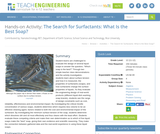Educational Use
Rating

Student teams are challenged to evaluate the design of several liquid soaps to answer the question, “Which soap is the best?” Through two simple teacher class demonstrations and the activity investigation, students learn about surface tension and how it is measured, the properties of surfactants (soaps), and how surfactants change the surface properties of liquids. As they evaluate the engineering design of real-world products (different liquid dish washing soap brands), students see the range of design constraints such as cost, reliability, effectiveness and environmental impact. By investigating the critical micelle concentration of various soaps, students determine which requires less volume to be an effective cleaning agent, factors related to both the cost and environmental impact of the surfactant. By investigating the minimum surface tension of the soap, students determine which dissolves dirt and oil most effectively and thus cleans with the least effort. Students evaluate these competing criteria and make their own determination as to which of five liquid soaps make the “best” soap, giving their own evidence and scientific reasoning. They make the connection between gathered data and the real-world experience in using these liquid soaps.

Subject:
Mathematics
Algebra
Measurement and Data
Physical Science
Physics
Material Type:
Activity/Lab
Provider:
TeachEngineering
Author:
Lauchlin Blue
Shawn Richard
02/07/2017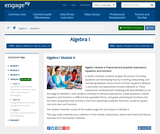Conditional Remix & Share Permitted
CC BY-NC-SA
Rating

In earlier modules, students analyze the process of solving equations and developing fluency in writing, interpreting, and translating between various forms of linear equations (Module 1) and linear and exponential functions (Module 3). These experiences combined with modeling with data (Module 2), set the stage for Module 4. Here students continue to interpret expressions, create equations, rewrite equations and functions in different but equivalent forms, and graph and interpret functions, but this time using polynomial functions, and more specifically quadratic functions, as well as square root and cube root functions.

Subject:
Algebra
Material Type:
Module
Provider:
New York State Education Department
Provider Set:
EngageNY
09/17/2013Unrestricted Use
CC BY
Rating

This is a task from the Illustrative Mathematics website that is one part of a complete illustration of the standard to which it is aligned. Each task has at least one solution and some commentary that addresses important asects of the task and its potential use. Here are the first few lines of the commentary for this task: It takes Clea 60 seconds to walk down an escalator when it is not operating, and only 24 seconds to walk down the escalator when it is operating. How m...

Subject:
Mathematics
Material Type:
Activity/Lab
Provider:
Illustrative Mathematics
Provider Set:
Illustrative Mathematics
Author:
Illustrative Mathematics
05/30/2013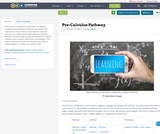Conditional Remix & Share Permitted
CC BY-NC
Rating

This course is designed to cover topics in Algebra ranging from polynomial, rational, and exponential functions to conic sections. Trigonometry concepts such as Law of Sines and Cosines will be introduced. Students will then begin analytic geometry and calculus concepts such as limits, derivatives, and integrals. This class is important for any student planning to take a college algebra or college pre-calculus class.

Subject:
Mathematics
Material Type:
Full Course
Author:
Deanna Mayers
06/10/2018Only Sharing Permitted
CC BY-NC-ND
Rating

This lesson unit is intended to help teachers assess how well students are able to use geometric properties to solve problems. In particular, it will help you identify and help students who have difficulty: decomposing complex shapes into simpler ones in order to solve a problem; bringing together several geometric concepts to solve a problem; and finding the relationship between radii of inscribed and circumscribed circles of right triangles.

Subject:
Geometry
Material Type:
Assessment
Lesson Plan
Provider:
Shell Center for Mathematical Education
U.C. Berkeley
Provider Set:
Mathematics Assessment Project (MAP)
04/26/2013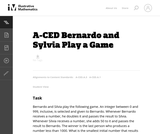Unrestricted Use
CC BY
Rating

This task presents a simple but mathematically interesting game whose solution is a challenging exercise in creating and reasoning with algebraic inequalities. The core of the task involves converting a verbal statement into a mathematical inequality in a context in which the inequality is not obviously presented, and then repeatedly using the inequality to deduce information about the structure of the game.

Subject:
Mathematics
Algebra
Material Type:
Activity/Lab
Provider:
Illustrative Mathematics
Provider Set:
Illustrative Mathematics
Author:
Illustrative Mathematics
10/21/2013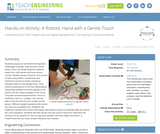Educational Use
Rating

Students groups act as NASA/GM engineers challenged to design, build and test robotic hands, which are tactile feedback systems made from cloth gloves and force sensor circuits. Student groups construct force sensor circuits using electric components and FlexiForce sensors to which resistance changes based on the applied force. They conduct experiments to find the mathematical relationship between the force applied to the sensor and the output voltages of the circuit. They take several measurements force vs. resistance, force vs. voltage and use the data to find the best fit curve models for the sensor. Different weights applied to the sensor are used as a scalable force. Students use traditional methods and current technology (calculators) to plot the collected data and define the curve equations. Students test their gloves and use a line of best fit to determine the minimum force required to crack an egg held between the index finger and thumb. A PowerPoint(TM) file and many student handouts are included.

Subject:
Engineering
Material Type:
Activity/Lab
Provider:
TeachEngineering
Provider Set:
TeachEngineering
Author:
Luis Avila
10/14/2015Unrestricted Use
CC BY
Rating

This resource is geared for teacher use. It is loosely linked to the Secondary Math II, Mathematics Vision Project curriculum.

Subject:
Mathematics
Material Type:
Unit of Study
Author:
Mindy Branson
08/18/2020Only Sharing Permitted
CC BY-NC-ND
Rating

This lesson unit is intended to help teachers assess how well students are able to: use the Pythagorean theorem to derive the equation of a circle; and translate between the geometric features of circles and their equations.

Subject:
Geometry
Material Type:
Assessment
Lesson Plan
Provider:
Shell Center for Mathematical Education
U.C. Berkeley
Provider Set:
Mathematics Assessment Project (MAP)
04/26/2013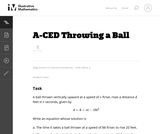Unrestricted Use
CC BY
Rating

Although this task is quite straightforward, it has a couple of aspects designed to encourage students to attend to the structure of the equation and the meaning of the variables in it. It fosters flexibility in seeing the same equation in two different ways, and it requires students to attend to the meaning of the variables in the preamble and extract the values from the descriptions.

Subject:
Mathematics
Algebra
Material Type:
Activity/Lab
Provider:
Illustrative Mathematics
Provider Set:
Illustrative Mathematics
Author:
Illustrative Mathematics